# (a) Identify three triangles in the figure. (b) Write the names of seven angles. (c) Write the names of six line segments (d) Which two triangles have ∠B as common?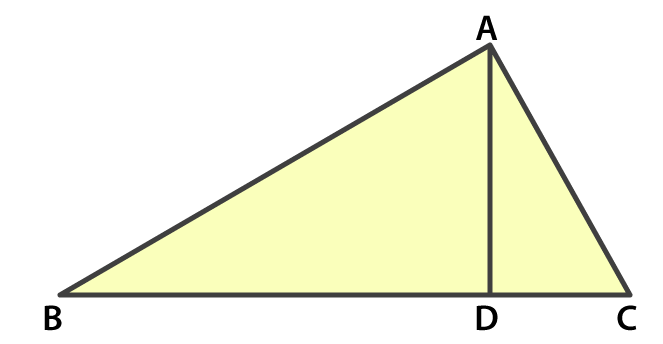Solution:

(a) The three triangles are ∠ABD, ∠ACB, ∠ADC

(c) The line segments are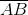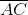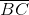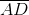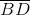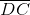(d) ∠ABD and ∠ABC are triangles which have ∠B as common.(84)(11)